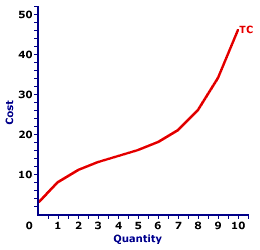Friday  December 1, 2023
 AmosWEB means Economics with a Touch of Whimsy!REAL GDP: The total market value, measured in constant prices, of all goods and services produced within the political boundaries of an economy during a given period of time, usually one year. The key is that real gross domestic product is measured in constant prices, the prices for a specific base year. Real gross domestic product, also termed constant gross domestic product, adjusts gross domestic product for inflation. You might want to compare real gross domestic product with the related term nominal GDP.TOTAL COST AND MARGINAL COST:

A mathematical connection between marginal cost and total cost stating that marginal cost IS the slope of the total cost curve. This relation between total cost and marginal cost is also seen with total variable cost. The slope of the total variable cost curve is marginal cost, as well. The relation between total cost and marginal cost is but another in the long line of applications of the total-marginal relation.
Total Cost and Marginal CostThe slope of the total cost curve (and total variable cost curve) is marginal cost. As such, if the total cost curve has a positive slope (that is, is upward sloping), then marginal cost is positive. Moreover, if the total cost curve has a positive and increasingly steeper slope, then the marginal cost is positive and rising. If the total cost curve has a positive and increasingly flatter slope, then the marginal cost is positive but falling.

This particular total-marginal relation applies to both total cost and total variable cost. Because not only is marginal cost the slope of the total cost curve, it is also the slope of the total variable cost curve. The reason is that any changes in total cost resulting from changing output is matched by changes in total variable cost. This occurs because total fixed cost is FIXED.

This two-paneled graph for the production of Wacky Willy Stuffed Amigos (those cute and cuddly armadillos and rattlesnakes) visually illustrates the connection between total cost and marginal cost. For the first few quantities of output (Stuffed Amigos), total cost in the top panel is positive AND the slope of the total cost curve decreases--it becomes flatter. This corresponds with a positive and decreasing marginal cost in the bottom panel up to 4 Stuffed Amigos. For the next several quantities of Stuffed Amigos output, the slope of the total cost curve becomes increasingly steeper. This corresponds to an increasing marginal cost in the bottom panel.

The prime conclusion is the key role played by the law of diminishing marginal returns in the slope of both the marginal cost curve and the total cost curve.

• The U-shape of the marginal cost curve is a direct reflection of first increasing marginal returns, as marginal cost falls to a minimum, then decreasing marginal returns and the onset of the law of diminishing marginal returns as marginal cost rises.

• However, because the marginal cost curve is a plot of the slope of the total cost curve, then the shape of the total cost curve also reflects the law of diminishing marginal returns. The flattening slope of the total cost curve for small quantities of output is due to increasing marginal returns. Then the onset of the law of diminishing marginal returns causes the total cost curve to become increasingly steeper.
While this diagram relates marginal cost and total cost, the same story applies to the relation between marginal cost and total variable cost. Marginal cost is also the slope of the total variable cost curve. As such, the shape of the total variable cost curve is also a reflection of increasing, then decreasing marginal returns.

 <= TOTAL COST TOTAL COST CURVE =>Recommended Citation:

TOTAL COST AND MARGINAL COST, AmosWEB Encyclonomic WEB*pedia, http://www.AmosWEB.com, AmosWEB LLC, 2000-2023. [Accessed: December 1, 2023].

Check Out These Related Terms...

Or For A Little Background...

And For Further Study...
Search Again?GREEN LOGIGUIN[What's This?] Today, you are likely to spend a great deal of time searching for a specialty store looking to buy either a birthday gift for your grandmother or a T-shirt commemorating yesterday. Be on the lookout for the last item on a shelf.Your Complete ScopeThe average length of a "business lunch" is about 36 minutes."Sometimes our light goes out, but is blown into flame by another human being. Each of us owes deepest thanks to those who have rekindled this light. "-- Albert Schweitzer, missionary physicianDOLDepartment of LaborA PEDestrian's Guide Xtra CreditTell us what you think about AmosWEB. Like what you see? Have suggestions for improvements? Let us know. Click the User Feedback link.| | | | | | | | | | |
| | | |

Thanks for visiting AmosWEB